# AP Physics C: Mechanics Practice Test 9

### Test Information11 questions15 minutes

1. A ball rolls horizontally with speed v off of a table a height h above the ground. Just before the ball hits the ground, what is its speed?

2.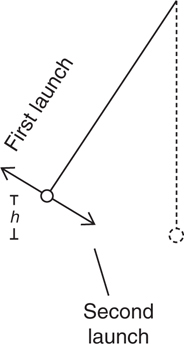A pendulum is launched into simple harmonic motion in two different ways, as shown above, from a point that is a height h above its lowest point. During both launches, the bob is given an initial speed of 3.0 m/s. On the first launch, the initial velocity of the bob is directed upward along the pendulum’s path, and on the second launch it is directed downward along the pendulum’s path. Which launch will cause the pendulum to swing with the larger amplitude?

3.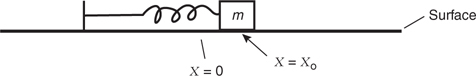The mass M is moving to the right with velocity v0 at position x = x0. Neglect friction. The spring has force constant k. What is the total mechanical energy of the block at this position?

4. A sphere, a cube, and a cylinder, all of equal mass, are released from rest from the top of a short incline. The surface of the incline is extremely slick, so much so that the objects do not rotate when released, but rather slide with negligible friction. Which reaches the base of the incline first?

5.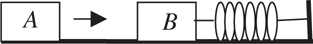Block B is at rest on a smooth tabletop. It is attached to a long spring, which is in turn anchored to the wall. Block A slides toward and collides with block B. Consider two possible collisions:

Collision I: Block A bounces back off of block B.

Collision II: Block A sticks to block B.

Which of the following is correct about the speed of block B immediately after the collision?

6.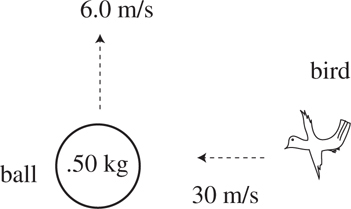A 0.30-kg bird is flying from right to left at 30 m/s. The bird collides with and sticks to a 0.50-kg ball that is moving straight up with speed 6.0 m/s. What is the magnitude of the momentum of the ball/bird combination immediately after collision?

7.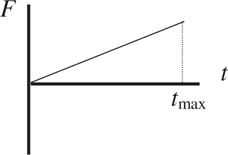The force F on a mass is shown above as a function of time t. Which of the following methods can be used to determine the impulse experienced by the mass?

I. multiplying the average force by tmax

II. calculating the area under the line on the graph

III. taking the integral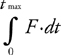8. A projectile is launched on level ground in a parabolic path so that its range would normally be 500 m. When the projectile is at the peak of its flight, the projectile breaks into two pieces of equal mass. One of these pieces falls straight down, with no further horizontal motion. How far away from the launch point does the other piece land?

9.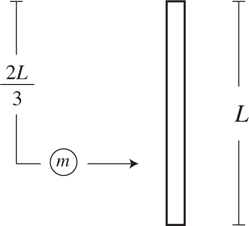A rigid rod of length L and mass M is floating at rest in space far from a gravitational field. A small blob of putty of mass m < M is moving to the right, as shown above. The putty hits and sticks to the rod a distance 2L/3 from the top end.

How will the rod/putty contraption move after the collision?

10.A rigid rod of length L and mass M is floating at rest in space far from a gravitational field. A small blob of putty of mass m < M is moving to the right, as shown above. The putty hits and sticks to the rod a distance 2L/3 from the top end.

What quantities are conserved in this collision?

11. A car rounds a banked curve of uniform radius. Three forces act on the car: a friction force between the tires and the road, the normal force from the road, and the weight of the car. Which provides the centripetal force which keeps the car in circular motion?